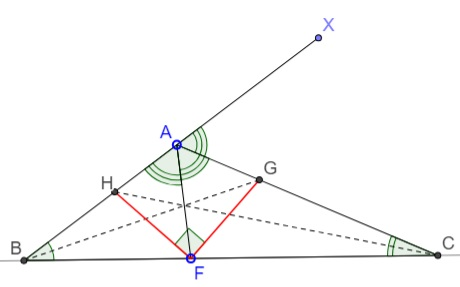# 60° Breeds 90°: What is this about? A Mathematical Droodle

ExplanationCopyright © 1996-2018 Alexander Bogomolny

The applet below is supposed to suggest the following problem:

Angle bisectors AF, BG, and CH in ΔABC are drawn. It is known that ∠A = 120°. Prove that angle GFH is right.Extend AB beyond A to, say, X. Since ∠BAC = 120°, ∠CAX = 60°. Also ∠CAF = 60°, so that AC is the external angle bisector at A of ΔABF. The external angle bisector at A intersects the (interior) angle bisector at B in an excenter of ΔABF. It follows that G is an excenter of that triangle. Another external angle bisector passes through G: that of the exterior angle at F. Therefore, FG is the bisector of ∠AFC. Similarly, FH is the bisector of ∠AFB. Since the two angles are supplementary, so that the angle between FG and FH is indeed 90°.

(Another solution has been posted elsewhere.)

### References

1. D. Fomin, A. Kirichenko, Leningrad Mathematical Olympiads 1987-1991, MathPro Press, 1994, pp. 38, 146-7### Related materialRead more...

• Midpoints of the Lines Joining In- and Excenters
• Circles Tangent to Circumcircle
• 60° Breeds 90°
• Cyclic Incenters
• Generalizing a Romanian Olympiad Problem
• Three Circles through the Incenter
• Circumcenter on Angle Bisector
• Euler's Formula and Poncelet Porism
•Copyright © 1996-2018 Alexander Bogomolny

 65264971